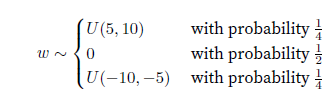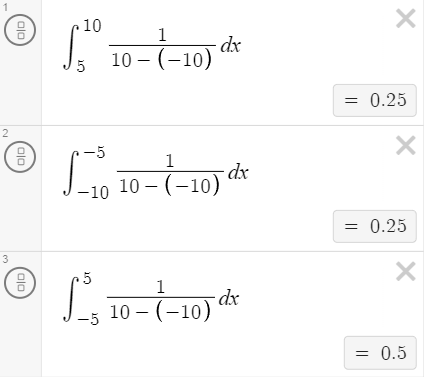# Parameter Management

For tied parameters (link), why is the gradient the sum of the gradients of the two layers? I was thinking it would be the product of the gradients of the two layers. Reasoning:

y = f(f(x))
dy/dx = f’(f(x))*f’(x) where x is a vector denoting the shared parameters.

(Cross posting from the D2L pytorch forum, since it does not really have anything to do with pytorch).

Hi @ganeshk, fantastic question! Even though it is not intuitively obvious, we design the operator by using “sum” rather than “product”. That aligns with the idea how we learn a convolution kernel. Check this tutorial for more details.

1 Like

This is helpful. Thanks. I suppose having a product is more likely to lead to problems like vanishing gradients. The sum should be more stable to that.

Great @ganeshk ! As you may understand now, theoretical intuition needs more practical experiments. Good luck!

Hi, is it possible to CustomInit weight parameter? Let’s say we have Con2D layer with size of 3x3 and in_channel = 1 = out_channel. I want to initialize weight to be np.arange(9). How to do it? Thank you.

Hi! Consider using `mxnet.init.Constant` initializer:

``````>>> from mxnet import gluon, init, np, npx
>>> npx.set_np()
>>> net = gluon.nn.Sequential()
>>> net
Sequential(
(0): Dense(-1 -> 1, linear)
)
>>> weights = np.ones(9)
>>> net.initialize(init=init.Constant(weights))
>>> output = net(np.random.uniform(size=(16, weights.shape)))
>>> net
Sequential(
(0): Dense(9 -> 1, linear)
)
``````

Also, remember that you can use `load_parameters` method to load the previously saved weights.

I have a doubt in the custom initialization section .How is the above initialization is implemented in the code given in the book:

``````def my_init(m):
if type(m) == nn.Linear:
print(
"Init",
*[(name, param.shape) for name, param in
m.named_parameters()])
nn.init.uniform_(m.weight, -10, 10)
m.weight.data *= m.weight.data.abs() >= 5
net.apply(my_init)
net.weight[:2]
``````

where is the probability applied in this code?

Hi, I am having problem understading the weight dimensions.

In Dense(8), shouldn’t the weight matrix have 4 rows and 8 columns and in Dense(1), 8 rows and 1 column? As shape of weight matrix is always num_inputs(rows) and num_outputs(columns)